# Tips From an Online Calculus Tutor: Stop Low-Dee-High, High-Dee-Low – Do This Instead

In Calculus 1 we learn several rules, tricks, and procedures to take the derivative of many types of functions. One of the more onerous to memorize and tedious to utilize is the “quotient rule.” The quotient rule is used for finding the derivate of a function that appears to demonstrate as two separate functions in the form of a fraction - sign up for our online calculus tutoring. That is, one function is being divided by another, so we are finding the derivative of this quotient. Here is an example of a function where you might use this rule:And here is the quotient rule itself: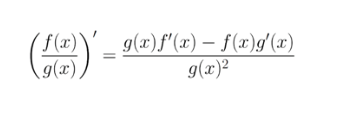Applied to our example above, f(x) would be 2x^4-6 since it is in the numerator, and g(x) would be x^2+7x+1 since it is in the denominator. Plugging into the quotient rule will, therefore, yield the following: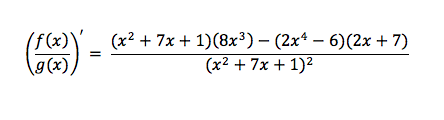At this point, we have a lot of distribution to do to try to simplify this. We’ll leave the bottom as is and do the top since nothing will cancel on the bottom: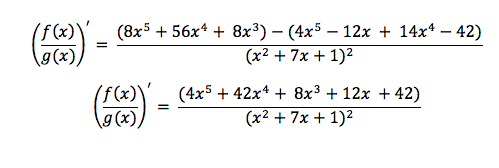And from here, we’re done. We could factor a ‘2’ out of the numerator, but that’s inconsequential at this point. So, what’s wrong with this process and the quotient rule? Here are three problems:

#### 1. It's obnoxious to memorize

Students often forget two things with the quotient rule: which term is subtracted from which, and which term goes on the bottom. It is also common to see students forget to square the bottom term. This is where the mnemonic comes in: “low d high minus high d low over low squared” (the “d” represents a derivative of either the “high” or “low” function).
This is a horrible mnemonic. Students frequently do “high d low minus low d high” when they mix it up since both ways sound fine. And the over low squared doesn’t fit at all – it just has to be memorized.

Some teachers come up with more of a story or song to make the mnemonic more memorable. Even when this is the case, we don’t want to be forced to rely on arbitrary memorizations to get through math when there is a better alternative: using the product rule.

#### 2. Answers tend to be harder to work with

Our example problem above was not an overly complicated function, but it did lead to a very large and complicated derivative. While it is one term, which can sometimes be desirable, it often makes things more difficult. It would be a lot more work if we needed to take a second or third derivative, or integrate to check our answer, or use long division to find skew asymptotes, or even to plug in numbers to test points or create a graph.
By using the product rule instead, we are more likely to get an answer that has simpler, separated terms that can be easier to work with in calculus.

#### 3. It's more work than it needs to be

In the quotient rule, we have a lot of distribution, a very large fraction, a complex formula to memorize, and a lot of algebra on our hands to simplify. It isn’t terrible, and certainly, many calculus students have gotten by just fine using it, but there is a method that is usually much simpler: using the product rule.

Now let’s see the solution to these problems.  Here’s the product rule for taking the derivative of two functions that are being multiplied: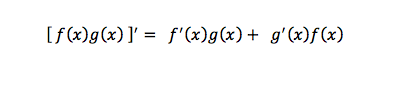The product rule is used very often and must be memorized for success in calculus. Luckily, it’s easy to remember, it doesn’t matter what order the terms are in, and it doesn’t require a mnemonic. However, it’s used for when we are multiplying two functions, so how can we use it instead of the quotient rule?

We can simply rewrite our fraction as a product. Using our same example from above: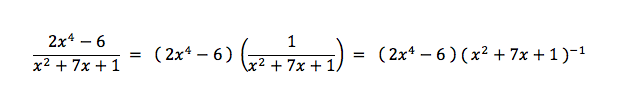Remember that we can reconstruct division as the multiplication of the reciprocal. This is the same as multiplying by our denominator to the power of negative one. Now we have two functions being multiplied, so we can use our product rule to find the derivative: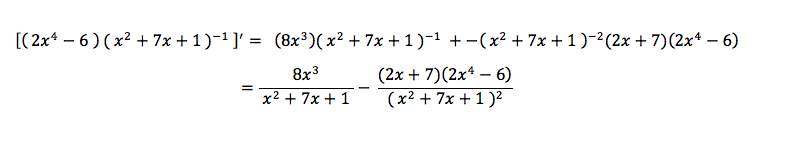Notice our answer does not look like the one we got using the quotient rule, but they are in fact algebraically equivalent. If we’d like, we can get the answer found above by simply using a common denominator and subtracting. Or, we can distribute and expand the terms even more.

The benefit of this is that we don’t have to memorize anything else that we’re just going to forget next year. We also get more practice with the product rule and the chain rule, the two most important rules for taking derivatives in early calculus. Instead of memorizing another mnemonic, we focus on mastering the skills we already know. We also end up doing less distribution and have an answer that is already partially separated in case we need to do more work with it.

The one downside of using the product rule instead of the quotient rule is the need to always use the chain rule with the product rule because of the extra negative one exponent on the denominator. But this is not a downside. You should have learned or are learning the chain rule already, and it is incredibly important for your success in calculus that you master it.

Practice your chain rule and product rule instead of memorizing an arbitrary rhyme in math class. You’ll be less confused, more confident, and ultimately do less work.